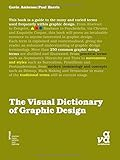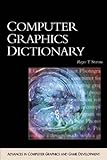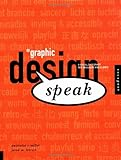Home: Graphic AcademyGraphics BooksGraphics File Formats Animation File Formats Bitmap File Formats CAD File Formats Cursor File Formats Font File Formats Icon File Formats Metafile File Formats Prepress File Formats Vector File Formats Video File Formats Web File Formats More Graphics File FormatsGraphics Software Banner Design Software CAD Software Diagram Drawing Software Illustration Software Photo Editing Software Video Conversion Software More Graphics SoftwareGraphics Tutorials Adobe Dreamweaver Tutorials Adobe Photoshop Tutorials AutoCAD Tutorials How To Draw Tutorials Banner Design Tutorial Banner Display Tutorial Color Combinations Tutorial Color-Safe Conversion Tutorial eBook Covers Tutorial Favicon Tutorial GIF + Photo Tutorial Good Web Design Image Slice Tutorial Image Tiling Tutorial Imagemap Tutorial Photography Tutorial Prevent Copying Tutorial Screenshots Tutorial Should I Use Flash? Text to Images Tutorial Web Image File Formats More Graphics TutorialsGraphics Glossary

 GraphicsAcademy.com  >  Glossary  >  Color Safe Palette

# Color Safe Palette

HTML allows web content to include page elements specified in 24 bit True Color. These can include page elements, pictures in True Color (such as PNGs and JPEGs), and pictures in formats with different palettes selected from True Color (such as GIFs).

Obviously, this could lead to a difficulties if the web page content includes more colors than be simultanously displayed on the user's screen (for example, many users may only a have 256 color screen). In these cases, web browsers typical attempt to dither any colors in the content which can not be displayed.

While dithering can often do a reasonable job of simulating more colors than can actually be displayed, it also can produce objectionable effects, particularly when displaying large areas of a solid color. For this reason, browsers on 256 color screens, define a standard palette, known as the "Color Safe Palette" - which consists of colors which will never be dithered. Thus, a web designer can design web pages and graphics using the Color Safe Palette and be assured that the colors will never be dithered.

The Color Safe Palette consists of 216 colors (the remaining 40 colors out of the 256 colors are reserved for system usage, and are different on different types of computer systems such as Apple Mac, Microsoft Windows, etc.).

Below is the color safe palette.

(In each cell, I have given the RGB values in the range 0 to 255, as well the hexadecimal notation used in HTML coding).

 #000000R=0 B=0 G=0 #000033R=0 B=51 G=0 #000066R=0 B=102 G=0 #000099R=0 B=153 G=0 #0000CCR=0 B=204 G=0 #0000FFR=0 B=255 G=0 #003300R=0 B=0 G=51 #003333R=0 B=51 G=51 #003366R=0 B=102 G=51 #003399R=0 B=153 G=51 #0033CCR=0 B=204 G=51 #0033FFR=0 B=255 G=51 #006600R=0 B=0 G=102 #006633R=0 B=51 G=102 #006666R=0 B=102 G=102 #006699R=0 B=153 G=102 #0066CCR=0 B=204 G=102 #0066FFR=0 B=255 G=102 #009900R=0 B=0 G=153 #009933R=0 B=51 G=153 #009966R=0 B=102 G=153 #009999R=0 B=153 G=153 #0099CCR=0 B=204 G=153 #0099FFR=0 B=255 G=153 #00CC00R=0 B=0 G=204 #00CC33R=0 B=51 G=204 #00CC66R=0 B=102 G=204 #00CC99R=0 B=153 G=204 #00CCCCR=0 B=204 G=204 #00CCFFR=0 B=255 G=204 #00FF00R=0 B=0 G=255 #00FF33R=0 B=51 G=255 #00FF66R=0 B=102 G=255 #00FF99R=0 B=153 G=255 #00FFCCR=0 B=204 G=255 #00FFFFR=0 B=255 G=255 #330000R=51 B=0 G=0 #330033R=51 B=51 G=0 #330066R=51 B=102 G=0 #330099R=51 B=153 G=0 #3300CCR=51 B=204 G=0 #3300FFR=51 B=255 G=0 #333300R=51 B=0 G=51 #333333R=51 B=51 G=51 #333366R=51 B=102 G=51 #333399R=51 B=153 G=51 #3333CCR=51 B=204 G=51 #3333FFR=51 B=255 G=51 #336600R=51 B=0 G=102 #336633R=51 B=51 G=102 #336666R=51 B=102 G=102 #336699R=51 B=153 G=102 #3366CCR=51 B=204 G=102 #3366FFR=51 B=255 G=102 #339900R=51 B=0 G=153 #339933R=51 B=51 G=153 #339966R=51 B=102 G=153 #339999R=51 B=153 G=153 #3399CCR=51 B=204 G=153 #3399FFR=51 B=255 G=153 #33CC00R=51 B=0 G=204 #33CC33R=51 B=51 G=204 #33CC66R=51 B=102 G=204 #33CC99R=51 B=153 G=204 #33CCCCR=51 B=204 G=204 #33CCFFR=51 B=255 G=204 #33FF00R=51 B=0 G=255 #33FF33R=51 B=51 G=255 #33FF66R=51 B=102 G=255 #33FF99R=51 B=153 G=255 #33FFCCR=51 B=204 G=255 #33FFFFR=51 B=255 G=255 #660000R=102 B=0 G=0 #660033R=102 B=51 G=0 #660066R=102 B=102 G=0 #660099R=102 B=153 G=0 #6600CCR=102 B=204 G=0 #6600FFR=102 B=255 G=0 #663300R=102 B=0 G=51 #663333R=102 B=51 G=51 #663366R=102 B=102 G=51 #663399R=102 B=153 G=51 #6633CCR=102 B=204 G=51 #6633FFR=102 B=255 G=51 #666600R=102 B=0 G=102 #666633R=102 B=51 G=102 #666666R=102 B=102 G=102 #666699R=102 B=153 G=102 #6666CCR=102 B=204 G=102 #6666FFR=102 B=255 G=102 #669900R=102 B=0 G=153 #669933R=102 B=51 G=153 #669966R=102 B=102 G=153 #669999R=102 B=153 G=153 #6699CCR=102 B=204 G=153 #6699FFR=102 B=255 G=153 #66CC00R=102 B=0 G=204 #66CC33R=102 B=51 G=204 #66CC66R=102 B=102 G=204 #66CC99R=102 B=153 G=204 #66CCCCR=102 B=204 G=204 #66CCFFR=102 B=255 G=204 #66FF00R=102 B=0 G=255 #66FF33R=102 B=51 G=255 #66FF66R=102 B=102 G=255 #66FF99R=102 B=153 G=255 #66FFCCR=102 B=204 G=255 #66FFFFR=102 B=255 G=255 #990000R=153 B=0 G=0 #990033R=153 B=51 G=0 #990066R=153 B=102 G=0 #990099R=153 B=153 G=0 #9900CCR=153 B=204 G=0 #9900FFR=153 B=255 G=0 #993300R=153 B=0 G=51 #993333R=153 B=51 G=51 #993366R=153 B=102 G=51 #993399R=153 B=153 G=51 #9933CCR=153 B=204 G=51 #9933FFR=153 B=255 G=51 #996600R=153 B=0 G=102 #996633R=153 B=51 G=102 #996666R=153 B=102 G=102 #996699R=153 B=153 G=102 #9966CCR=153 B=204 G=102 #9966FFR=153 B=255 G=102 #999900R=153 B=0 G=153 #999933R=153 B=51 G=153 #999966R=153 B=102 G=153 #999999R=153 B=153 G=153 #9999CCR=153 B=204 G=153 #9999FFR=153 B=255 G=153 #99CC00R=153 B=0 G=204 #99CC33R=153 B=51 G=204 #99CC66R=153 B=102 G=204 #99CC99R=153 B=153 G=204 #99CCCCR=153 B=204 G=204 #99CCFFR=153 B=255 G=204 #99FF00R=153 B=0 G=255 #99FF33R=153 B=51 G=255 #99FF66R=153 B=102 G=255 #99FF99R=153 B=153 G=255 #99FFCCR=153 B=204 G=255 #99FFFFR=153 B=255 G=255 #CC0000R=204 B=0 G=0 #CC0033R=204 B=51 G=0 #CC0066R=204 B=102 G=0 #CC0099R=204 B=153 G=0 #CC00CCR=204 B=204 G=0 #CC00FFR=204 B=255 G=0 #CC3300R=204 B=0 G=51 #CC3333R=204 B=51 G=51 #CC3366R=204 B=102 G=51 #CC3399R=204 B=153 G=51 #CC33CCR=204 B=204 G=51 #CC33FFR=204 B=255 G=51 #CC6600R=204 B=0 G=102 #CC6633R=204 B=51 G=102 #CC6666R=204 B=102 G=102 #CC6699R=204 B=153 G=102 #CC66CCR=204 B=204 G=102 #CC66FFR=204 B=255 G=102 #CC9900R=204 B=0 G=153 #CC9933R=204 B=51 G=153 #CC9966R=204 B=102 G=153 #CC9999R=204 B=153 G=153 #CC99CCR=204 B=204 G=153 #CC99FFR=204 B=255 G=153 #CCCC00R=204 B=0 G=204 #CCCC33R=204 B=51 G=204 #CCCC66R=204 B=102 G=204 #CCCC99R=204 B=153 G=204 #CCCCCCR=204 B=204 G=204 #CCCCFFR=204 B=255 G=204 #CCFF00R=204 B=0 G=255 #CCFF33R=204 B=51 G=255 #CCFF66R=204 B=102 G=255 #CCFF99R=204 B=153 G=255 #CCFFCCR=204 B=204 G=255 #CCFFFFR=204 B=255 G=255 #FF0000R=255 B=0 G=0 #FF0033R=255 B=51 G=0 #FF0066R=255 B=102 G=0 #FF0099R=255 B=153 G=0 #FF00CCR=255 B=204 G=0 #FF00FFR=255 B=255 G=0 #FF3300R=255 B=0 G=51 #FF3333R=255 B=51 G=51 #FF3366R=255 B=102 G=51 #FF3399R=255 B=153 G=51 #FF33CCR=255 B=204 G=51 #FF33FFR=255 B=255 G=51 #FF6600R=255 B=0 G=102 #FF6633R=255 B=51 G=102 #FF6666R=255 B=102 G=102 #FF6699R=255 B=153 G=102 #FF66CCR=255 B=204 G=102 #FF66FFR=255 B=255 G=102 #FF9900R=255 B=0 G=153 #FF9933R=255 B=51 G=153 #FF9966R=255 B=102 G=153 #FF9999R=255 B=153 G=153 #FF99CCR=255 B=204 G=153 #FF99FFR=255 B=255 G=153 #FFCC00R=255 B=0 G=204 #FFCC33R=255 B=51 G=204 #FFCC66R=255 B=102 G=204 #FFCC99R=255 B=153 G=204 #FFCCCCR=255 B=204 G=204 #FFCCFFR=255 B=255 G=204 #FFFF00R=255 B=0 G=255 #FFFF33R=255 B=51 G=255 #FFFF66R=255 B=102 G=255 #FFFF99R=255 B=153 G=255 #FFFFCCR=255 B=204 G=255 #FFFFFFR=255 B=255 G=255

### Discuss Color Safe Palette

Related Pages on this site:

Related Books:
• A Visual Dictionary of Graphic Design

Disclosure: Products details and descriptions provided by Amazon.com. Our company may receive a payment if you purchase products from them after following a link from this website.By Gavin AmbroseBrand: Fairchild BooksReleased: 2006-11-15Paperback (288 pages)Used Book in Good ConditionProduct Description:The Visual Dictionary of Graphic Design is a comprehensive guide to the numerous terms used within graphic design and associated disciplines.Over 250 terms are explained and contextualized, with concise definitions accompanied by illustrations and examples taken from historical and contemporary graphic design.The dictionary covers traditional practice and process terms as well as modern terminology. It also defines a wide variety of practical terms, such as Perfect binding, Deboss and Strikethrough, as well as movements and styles including Surrealism, Psychadelia and Postmodernism.List Price: \$22.95*Lowest New Price: \$16.66*Lowest Used Price: \$1.97*Usually ships in 1-2 business days**(As of 16:32 Pacific 21 Dec 2019 More Info)Buy It Now

• Computer Graphics Dictionary (ADVANCES IN COMPUTER GRAPHICS AND GAME DEVELOPMENT SERIES)

Disclosure: Products details and descriptions provided by Amazon.com. Our company may receive a payment if you purchase products from them after following a link from this website.By Roger StevensBrand: Charles River MediaHardcover (460 pages)Used Book in Good ConditionProduct Description:The popularity of special effects movies and the video game industry has introduced thousands of new terms, techniques, and software applications to both the computing professional and the general user. Unfortunately, these graphics creations represent a convergence of expertise from several different subject areas, resulting in a lack of familiarity with many terms. This unique dictionary includes over 4,000 definitions and illustrations from the computer graphics world, including those from digital filmmaking, animation, programming, mathematics, holography, photorealism, lighting, character creation, game development, modeling, and more.List Price: \$49.95*Lowest New Price: \$44.70*Lowest Used Price: \$4.54*Usually ships in 24 hours**(As of 16:32 Pacific 21 Dec 2019 More Info)Buy It Now

• Graphic Design Speak: A Visual Dictionary for Designers and Clients

Disclosure: Products details and descriptions provided by Amazon.com. Our company may receive a payment if you purchase products from them after following a link from this website.By Anistatia MillerBrand: Rockport PublishersHardcover (160 pages)Used Book in Good ConditionProduct Description:What does a client or art director really mean when they say "I want a clean look" or "The design should be bold"? Graphic Design Speak offers the answers in an easy-to-follow format that both clients and designers can use as points of reference in design briefs or strategy meetings. This book is an essential tool for any designer seeking to gain a better knowledge of the wants and needs of their clients. --Over 200 color projects showing specific design solutions to answer client demands --An international gathering of designers, studios, and ad agencies --Quotes, tips, and anecdotes from both designers and clientsList Price: \$40.00*Lowest New Price: \$20.85*Lowest Used Price: \$4.40**(As of 16:32 Pacific 21 Dec 2019 More Info)Buy It NowDisclosure: Our company's websites' content (including this website's content) includes advertisements for our own company's websites, products, and services, and for other organization's websites, products, and services. In the case of links to other organization's websites, our company may receive a payment, (1) if you purchase products or services, or (2) if you sign-up for third party offers, after following links from this website. Unless specifically otherwise stated, information about other organization's products and services, is based on information provided by that organization, the product/service vendor, and/or publicly available information - and should not be taken to mean that we have used the product/service in question. Additionally, our company's websites contain some adverts which we are paid to display, but whose content is not selected by us, such as Google AdSense ads. For more detailed information, please see Advertising/Endorsements Disclosures Our sites use cookies, some of which may already be set on your computer. Use of our site constitutes consent for this. For details, please see Privacy. Click privacy for information about our company's privacy, data collection and data retention policies, and your rights. Contact Us     Privacy     Terms Of Use     Advertising/Endorsements Disclosures Copyright © 2005-2018, Answers 2000 Limited In Association With Amazon.comAnswers 2000 Limited is a participant in the Amazon Services LLC Associates Program, an affiliate advertising program designed to provide a means for sites to earn advertising fees by advertising and linking to Amazon.com.In Association With Amazon.co.ukAnswers 2000 Limited is a participant in the Amazon EU Associates Programme, an affiliate advertising programme designed to provide a means for sites to earn advertising fees by advertising and linking to Amazon.co.uk.As an Amazon Associate, our company earns from qualifying purchases. Amazon, the Amazon logo, Endless, and the Endless logo are trademarks of Amazon.com, Inc. or its affiliates.CERTAIN CONTENT THAT APPEARS ON THIS SITE COMES FROM AMAZON SERVICES LLC. THIS CONTENT IS PROVIDED 'AS IS' AND IS SUBJECT TO CHANGE OR REMOVAL AT ANY TIME.CERTAIN CONTENT THAT APPEARS ON THIS SITE,COMES FROM AMAZON EU S.ą r.l. THIS CONTENT IS PROVIDED 'AS IS' AND IS SUBJECT TO CHANGE OR REMOVAL AT ANY TIME. All trademarks are property of their respective owners. All third party content and adverts are copyright of their respective owners. Some graphics on our web sites are Copyright (C) 1997-2000 Hemera Technologies Inc., and used under license. All such pictures are provided for viewing purposes only and are not to be saved or downloaded. All such pictures of recognizable individuals are models and used for illustrative purposes only, and not meant to imply any association or endorsement of said individual with any product or service.